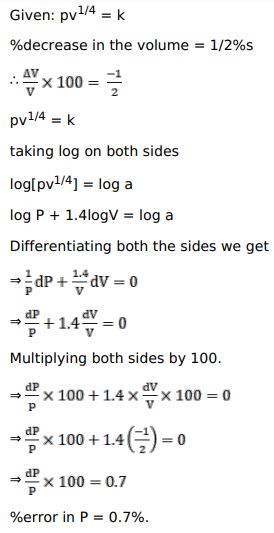# Solve this following

Question:

The pressure $\mathrm{p}$ and the volume $\mathrm{V}$ of a gas are connected by the relation, $\mathrm{p} \mathrm{V}^{1 / 4}=\mathrm{k}$, where $\mathrm{k}$ is a constant. Find the percentage increase in the pressure, corresponding to a diminution of $0.5 \%$ in the volume.

Solution: